# Greedy Optimal Homotopy and Homology Generators Jeff Erickson

• Slides: 36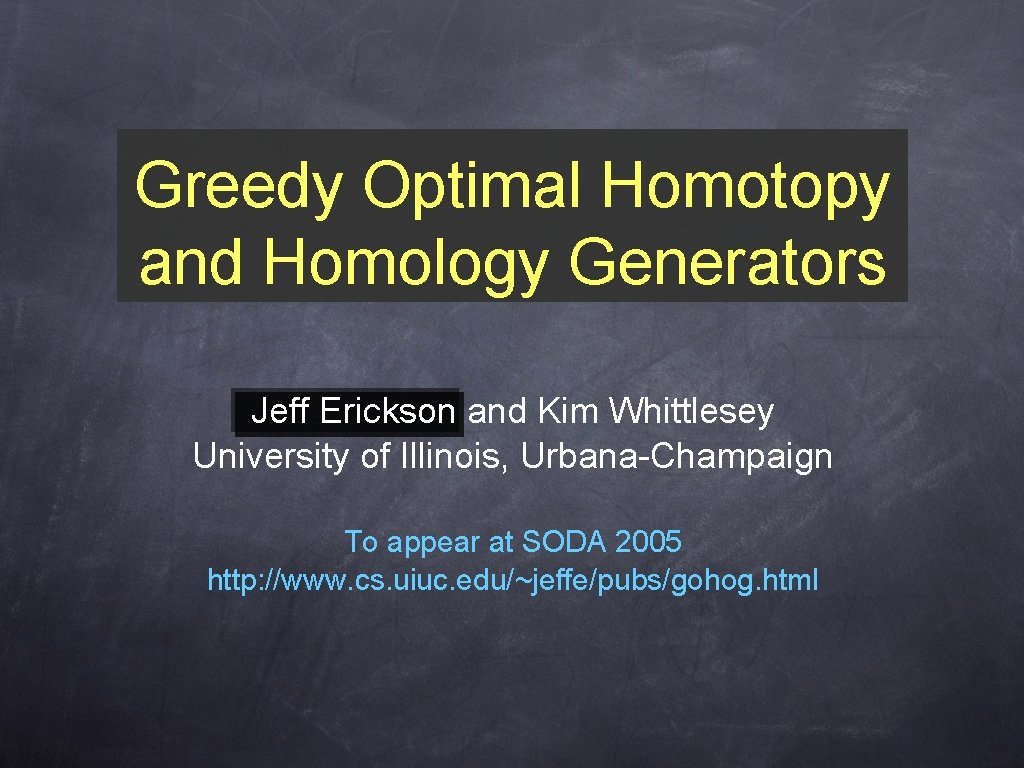Greedy Optimal Homotopy and Homology Generators Jeff Erickson and Kim Whittlesey University of Illinois, Urbana-Champaign To appear at SODA 2005 http: //www. cs. uiuc. edu/~jeffe/pubs/gohog. html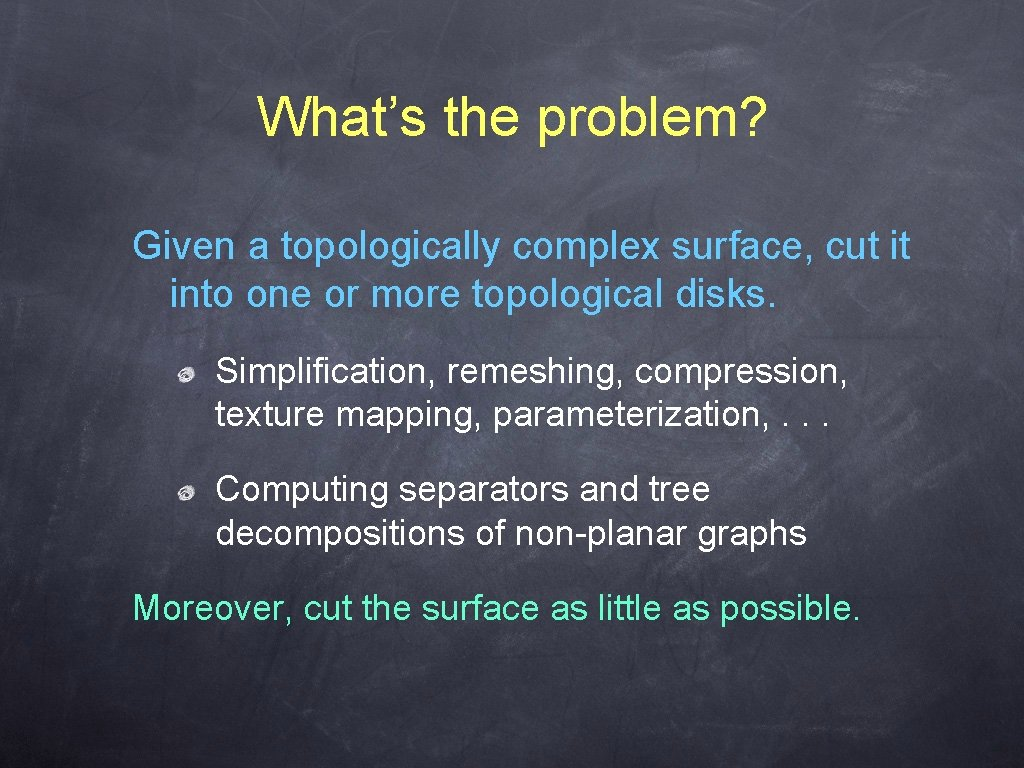What’s the problem? Given a topologically complex surface, cut it into one or more topological disks. Simplification, remeshing, compression, texture mapping, parameterization, . . . Computing separators and tree decompositions of non-planar graphs Moreover, cut the surface as little as possible.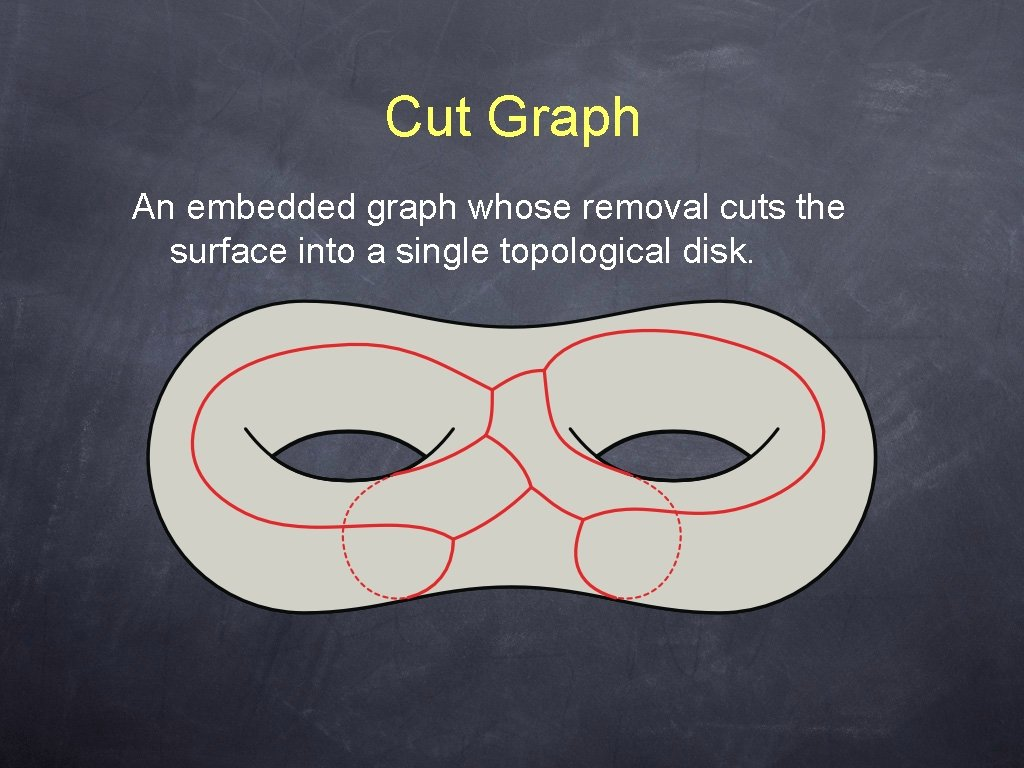Cut Graph An embedded graph whose removal cuts the surface into a single topological disk.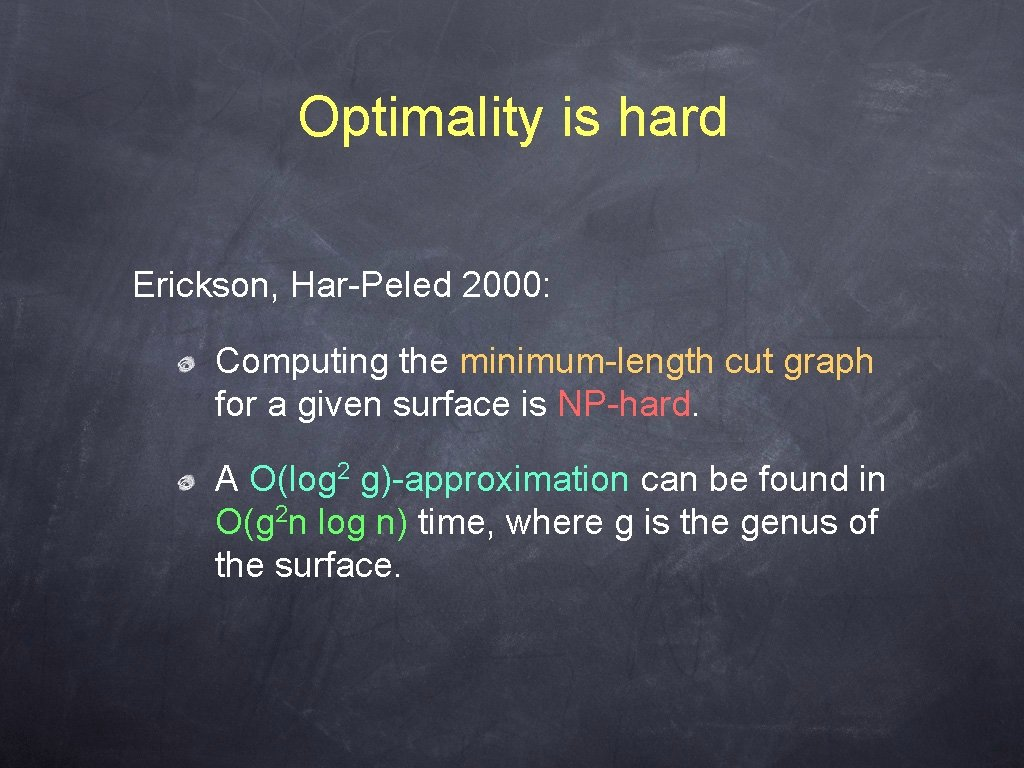Optimality is hard Erickson, Har-Peled 2000: Computing the minimum-length cut graph for a given surface is NP-hard. A O(log 2 g)-approximation can be found in O(g 2 n log n) time, where g is the genus of the surface.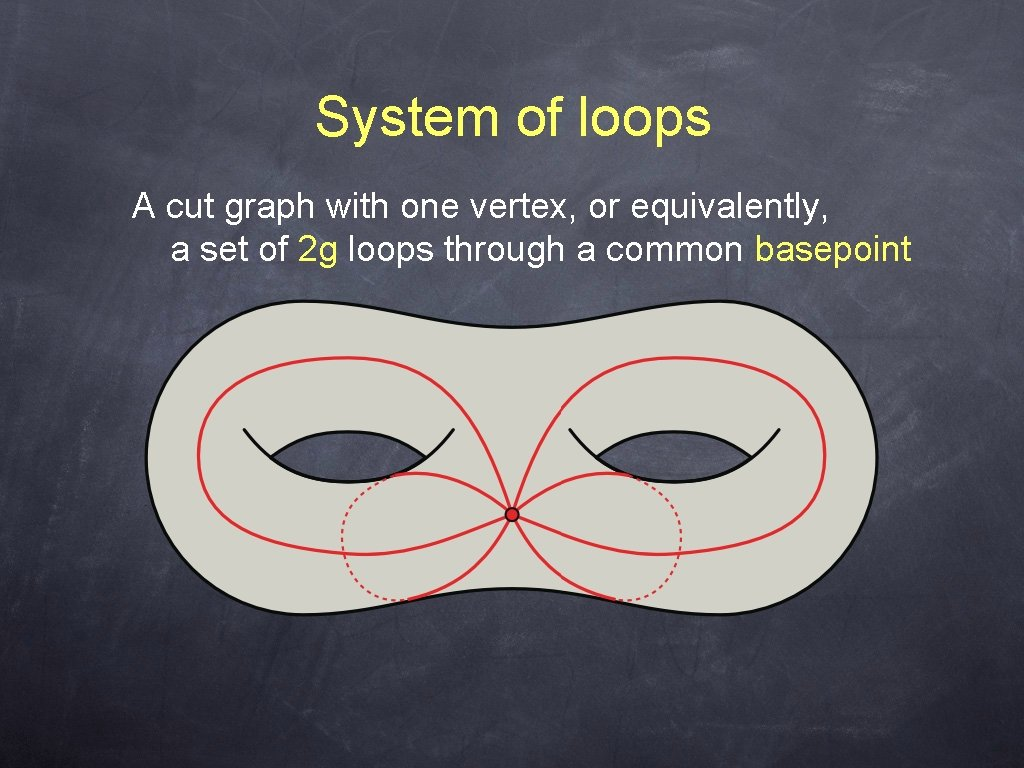System of loops A cut graph with one vertex, or equivalently, a set of 2 g loops through a common basepointOptimality is not so hard? Colin de Verdière, Lazarus 2002: Given a system of loops on a surface, the shortest system of loops in the same homotopy class can be computed in (large) polynomial time. * *under some mild (and probably unnecessary) assumptions.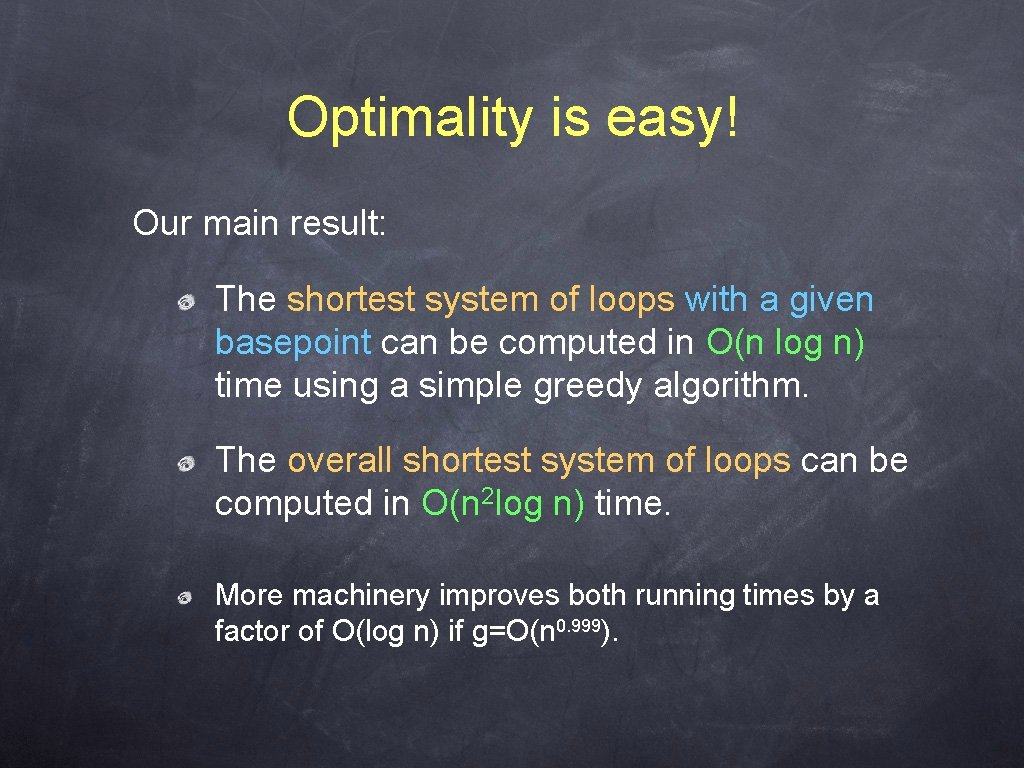Optimality is easy! Our main result: The shortest system of loops with a given basepoint can be computed in O(n log n) time using a simple greedy algorithm. The overall shortest system of loops can be computed in O(n 2 log n) time. More machinery improves both running times by a factor of O(log n) if g=O(n 0. 999).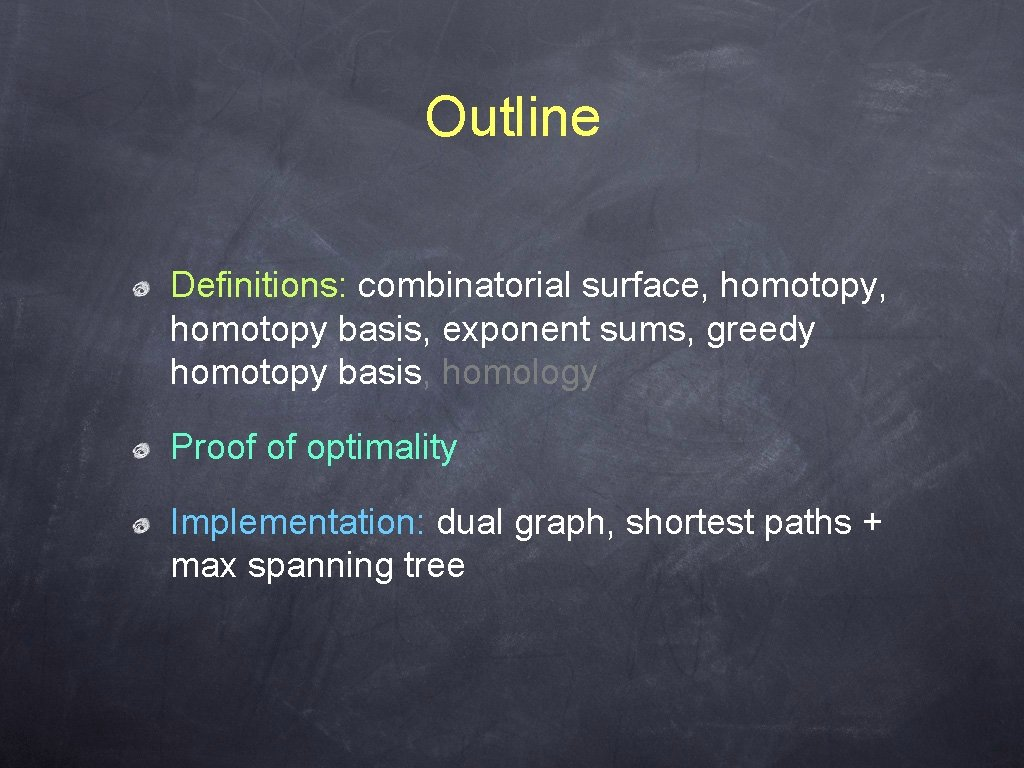Outline Definitions: combinatorial surface, homotopy basis, exponent sums, greedy homotopy basis, homology Proof of optimality Implementation: dual graph, shortest paths + max spanning treeSurfaces and topology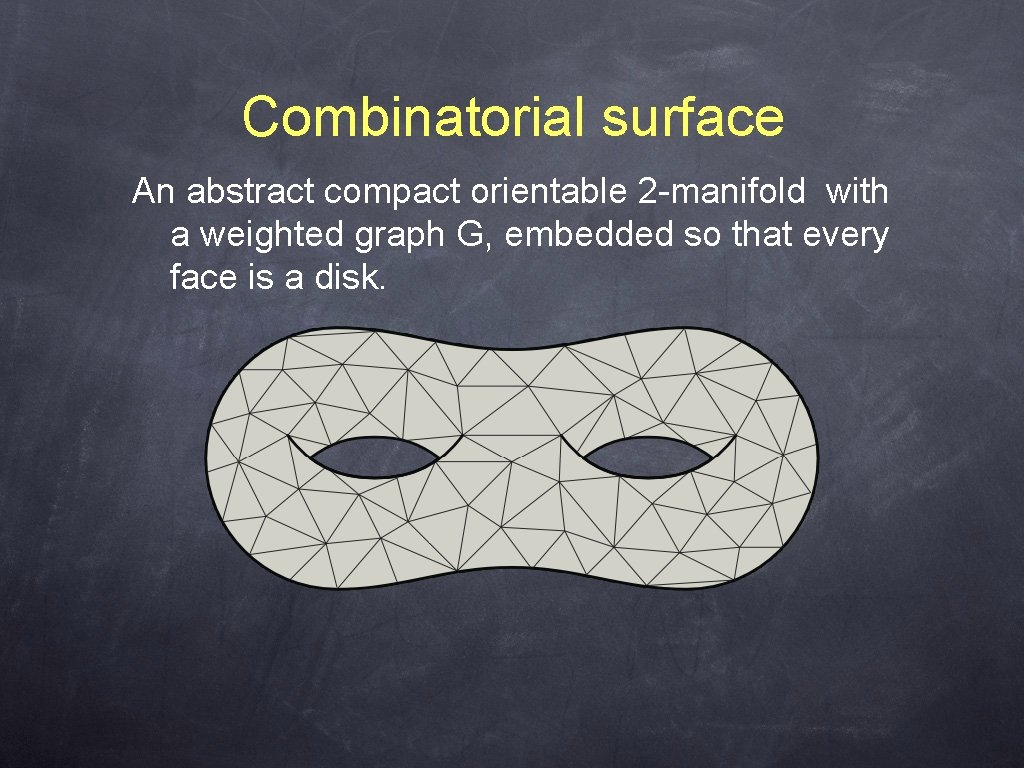Combinatorial surface An abstract compact orientable 2 -manifold with a weighted graph G, embedded so that every face is a disk.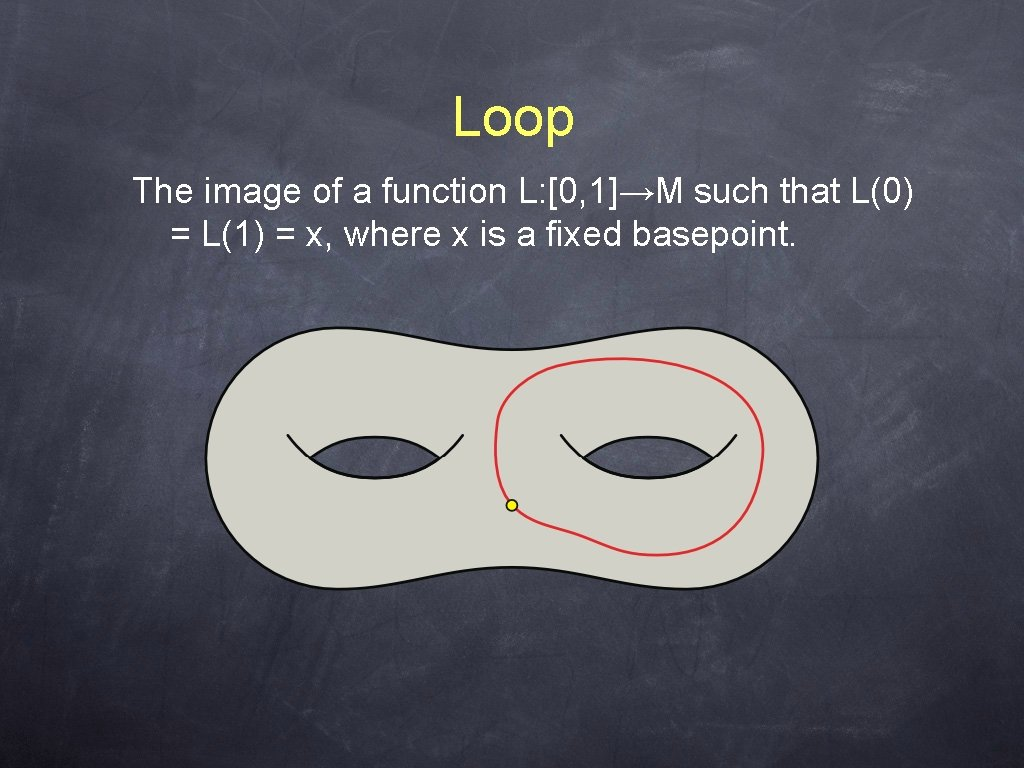Loop The image of a function L: [0, 1]→M such that L(0) = L(1) = x, where x is a fixed basepoint.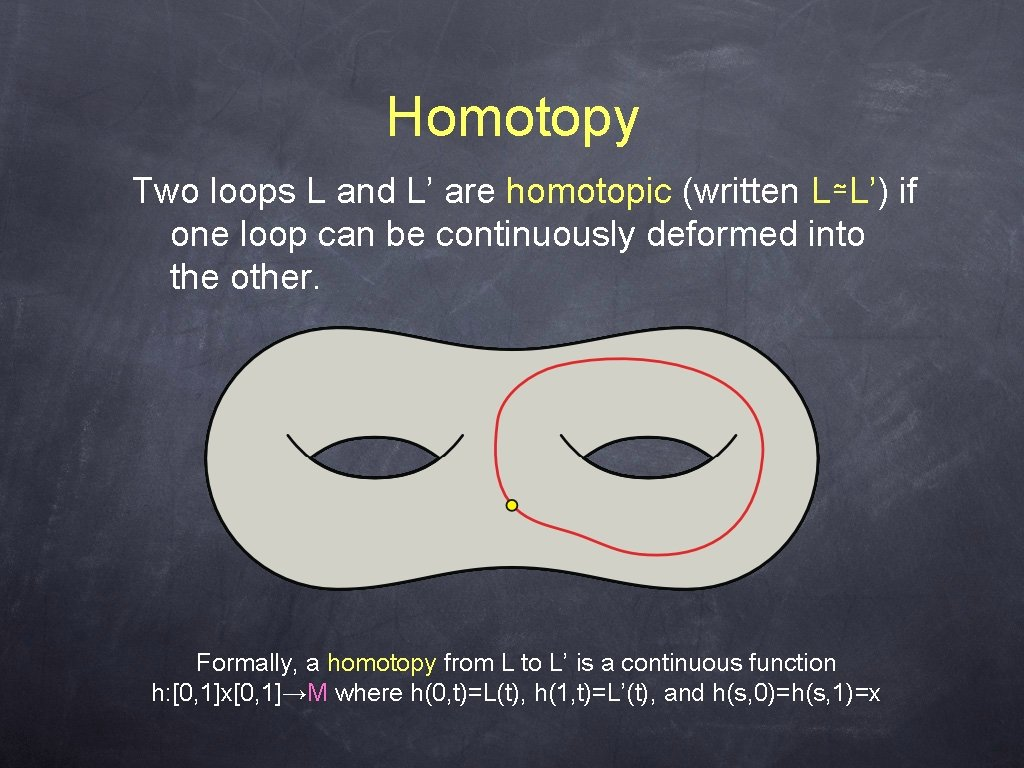Homotopy Two loops L and L’ are homotopic (written L≃L’) if one loop can be continuously deformed into the other. Formally, a homotopy from L to L’ is a continuous function h: [0, 1]x[0, 1]→M where h(0, t)=L(t), h(1, t)=L’(t), and h(s, 0)=h(s, 1)=x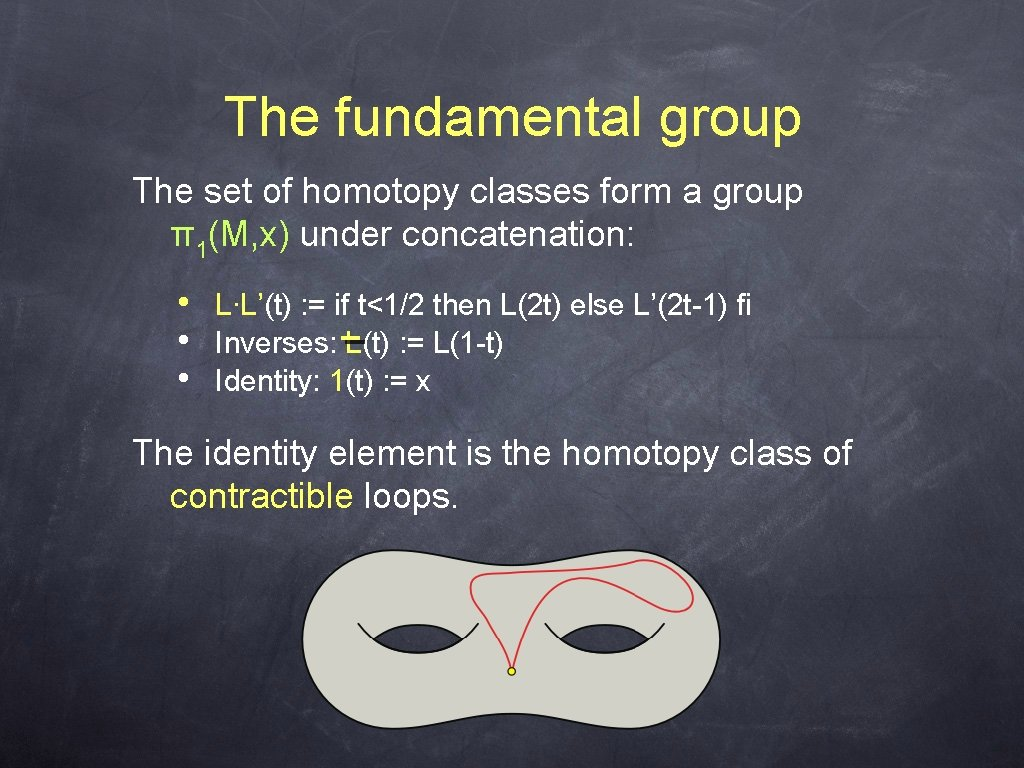The fundamental group The set of homotopy classes form a group π1(M, x) under concatenation: • • • L∙L’(t) : = if t<1/2 then L(2 t) else L’(2 t-1) fi Inverses: L(t) : = L(1 -t) Identity: 1(t) : = x The identity element is the homotopy class of contractible loops.Homotopy basis 2 g loops α 1, α 2, . . . , α 2 g that generate π1(M, x): Every loop is homotopic to a concatenation of basis loops and/or their inverses. Every system of loops is a homotopy basis, but not vice versa!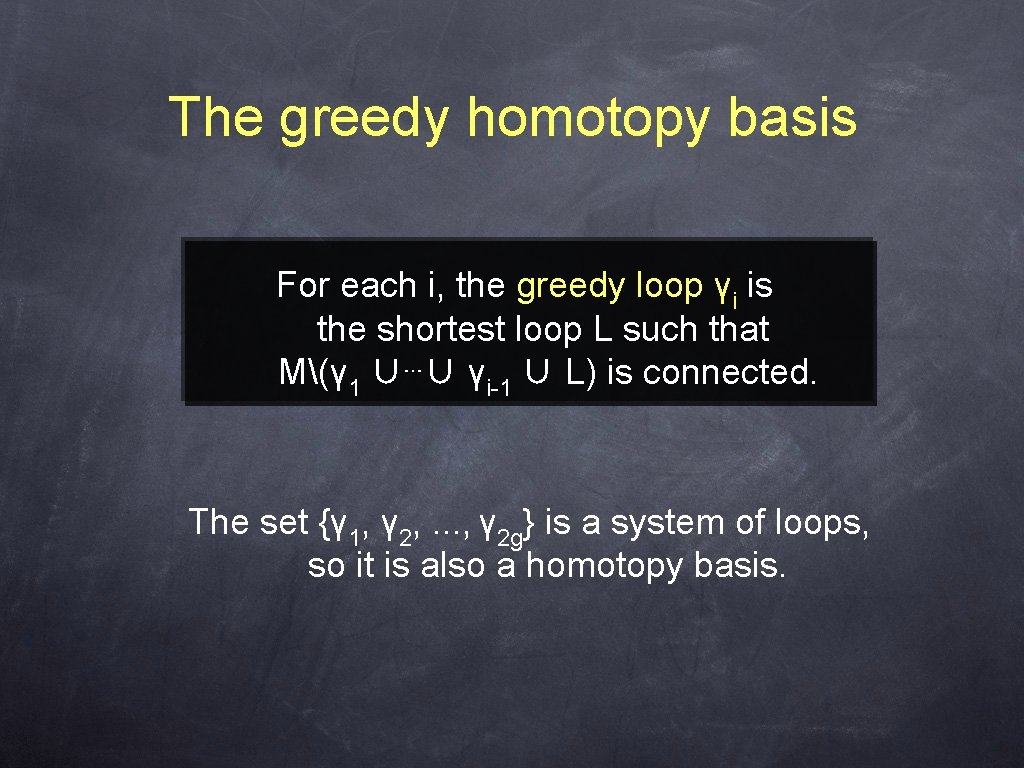The greedy homotopy basis For each i, the greedy loop γi is the shortest loop L such that M(γ 1 ∪⋯∪ γi-1 ∪ L) is connected. The set {γ 1, γ 2, . . . , γ 2 g} is a system of loops, so it is also a homotopy basis.Proof of optimalityShortest loops Let σ(uv) denote the shortest loop in G that contains the edge uv; this loop consists of 1. the shortest path from x to u, 2. the edge uv, and 3. the shortest path from v to x.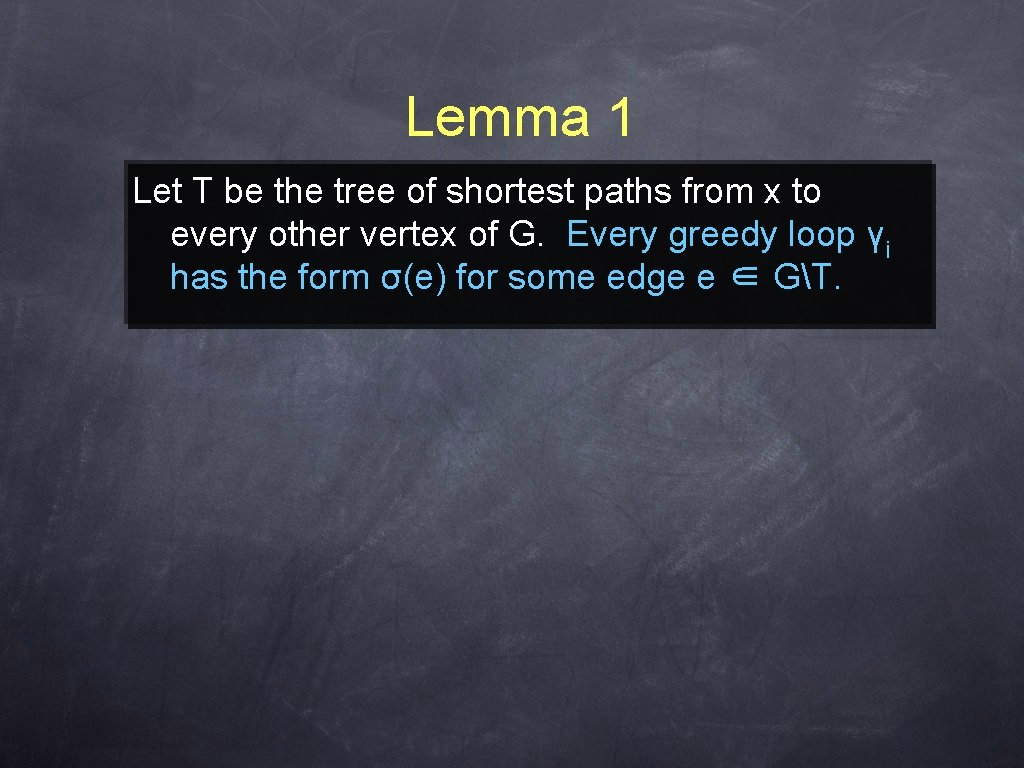Lemma 1 Let T be the tree of shortest paths from x to every other vertex of G. Every greedy loop γi has the form σ(e) for some edge e ∈ GT.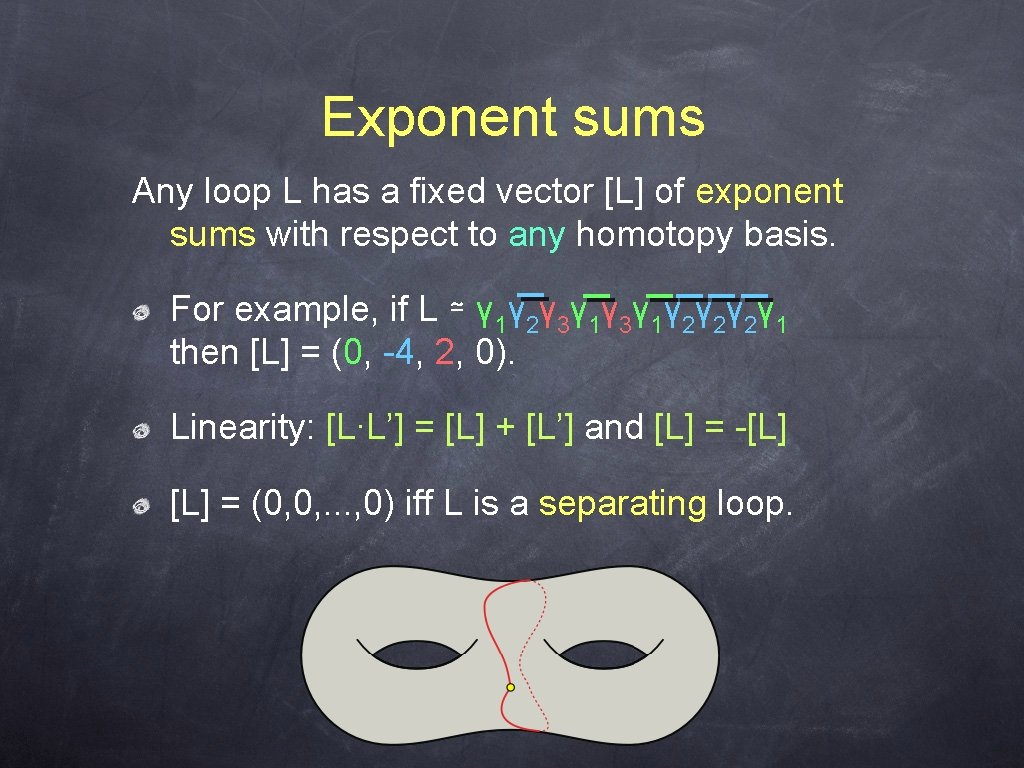Exponent sums Any loop L has a fixed vector [L] of exponent sums with respect to any homotopy basis. For example, if L ≃ γ 1γ 2γ 3γ 1γ 2γ 2γ 2γ 1 then [L] = (0, -4, 2, 0). Linearity: [L∙L’] = [L] + [L’] and [L] = -[L] = (0, 0, . . . , 0) iff L is a separating loop.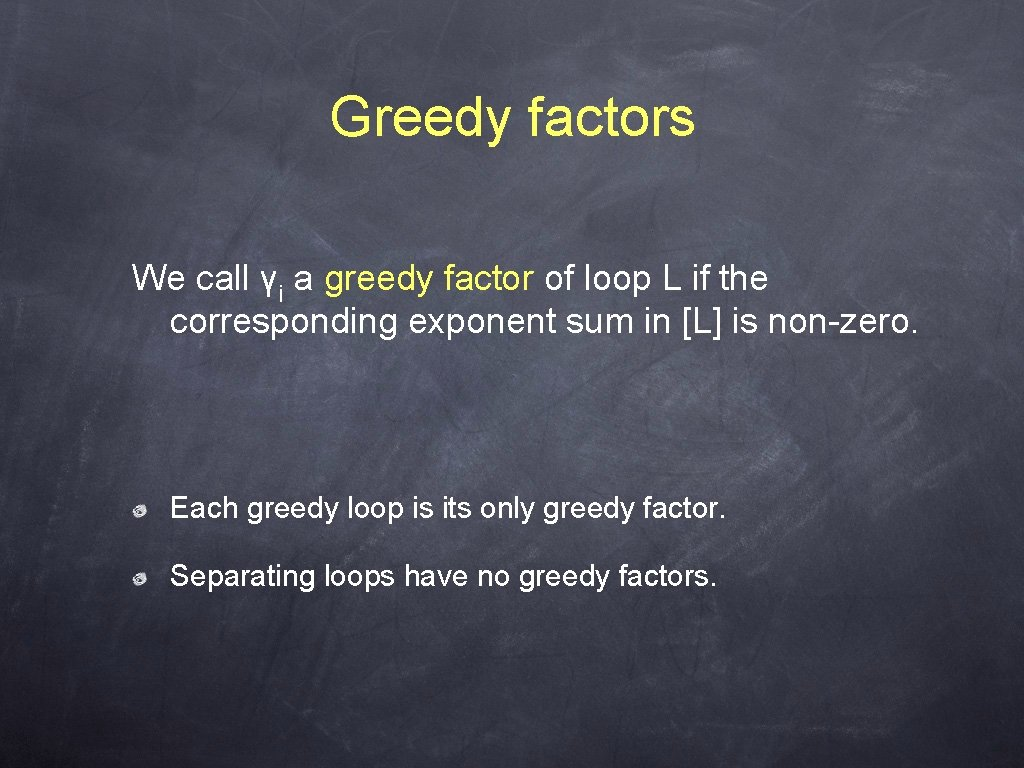Greedy factors We call γi a greedy factor of loop L if the corresponding exponent sum in [L] is non-zero. Each greedy loop is its only greedy factor. Separating loops have no greedy factors.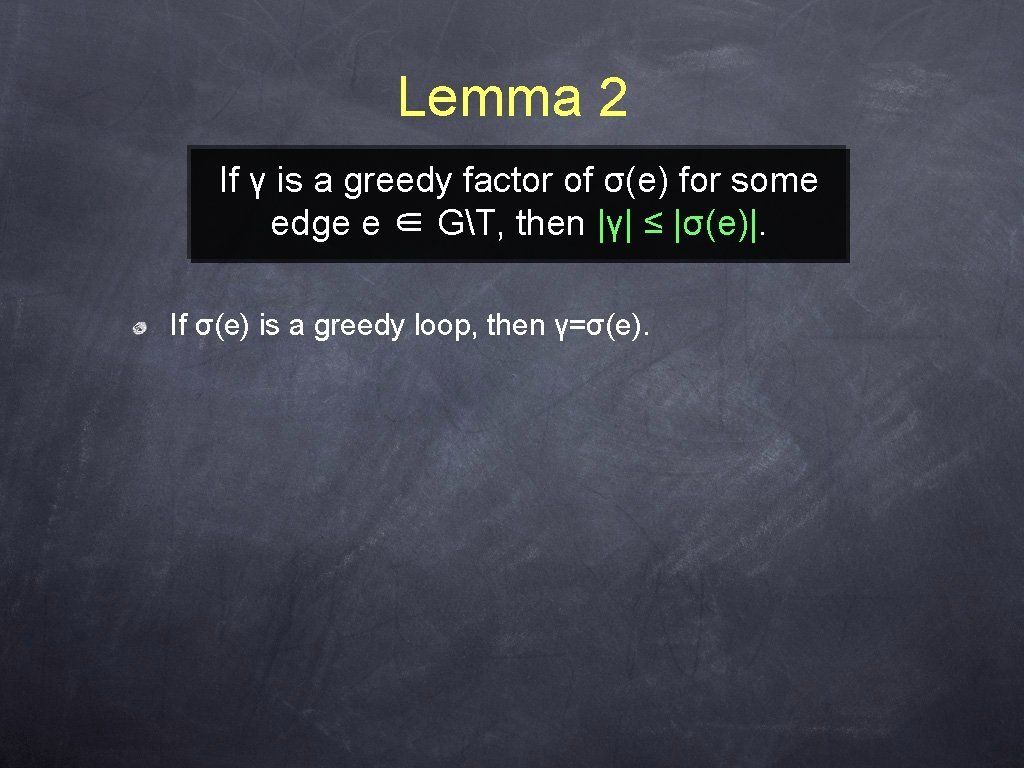Lemma 2 If γ is a greedy factor of σ(e) for some edge e ∈ GT, then |γ| ≤ |σ(e)|. If σ(e) is a greedy loop, then γ=σ(e).Lemma 2 If γ is a greedy factor of σ(e) for some edge e ∈ GT, then |γ| ≤ |σ(e)|. Let γ 1, γ 2, . . . , γi be the greedy loops shorter than σ(e). By definition, M(γ 1 ∪ ⋯ ∪ γi ∪ σ(e)) is disconnected. σ(e) γ 3 γ 1 γ 2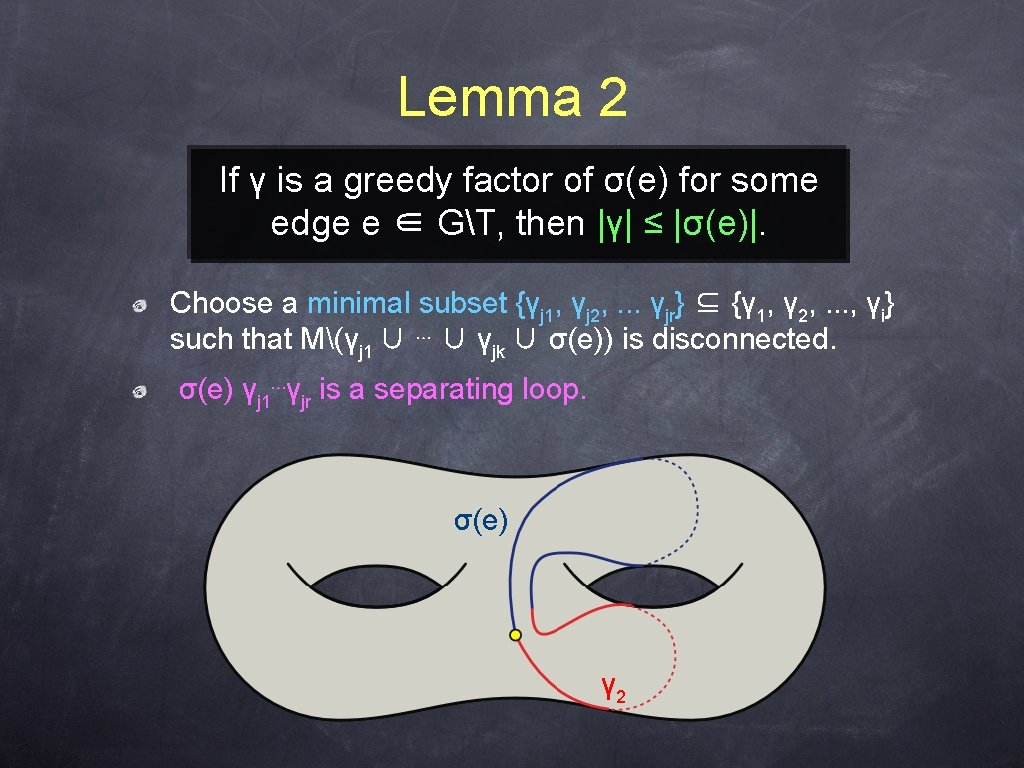Lemma 2 If γ is a greedy factor of σ(e) for some edge e ∈ GT, then |γ| ≤ |σ(e)|. Choose a minimal subset {γj 1, γj 2, . . . γjr} ⊆ {γ 1, γ 2, . . . , γi} such that M(γj 1 ∪ ⋯ ∪ γjk ∪ σ(e)) is disconnected. σ(e) γj 1⋯γjr is a separating loop. σ(e) γ 2Lemma 2 If γ is a greedy factor of σ(e) for some edge e ∈ GT, then |γ| ≤ |σ(e)|. [σ(e) γj 1⋯γjr] = (0, 0, . . . 0), so [σ(e)] = - [γj 1] - ⋯ - [γjr]. Thus γj 1, γj 2, . . . , γjr are the only greedy factors of σ(e) γ 2Lemma 3 If γ is a greedy factor of any loop L, then |γ| ≤ |L|. Let e 1, e 2, . . . , er be the sequence of edges in GT traversed by L. L ≃ σ(e 1)⋅σ(e 2)⋯σ(er). If γ is a greedy factor of L, then for some i, γ is a greedy factor of σ(ei), so |γ| ≤ |σ(ei)|. By definition, |σ(ei)| ≤ |L| for all i.Lemma 4 Any homotopy basis {α 1, α 2, . . . , α 2 g} can be reordered so that γi is a greedy factor of αi for all i. There is a nonsingular linear transformation mapping [L]α to [L]γ. The ith column of the transformation matrix is the vector [αi]γ.Main Theorem Any homotopy basis {α 1, α 2, . . . , α 2 g} can be reordered so that |γi| ≤ |αi| for all i.The Algorithm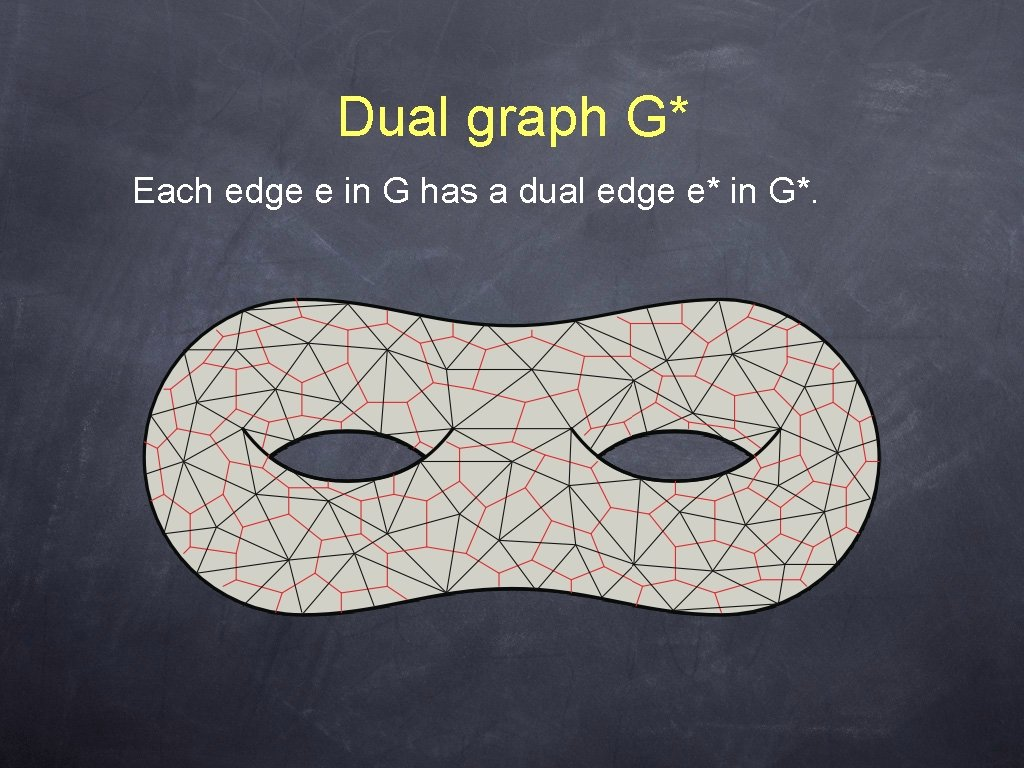Dual graph G* Each edge e in G has a dual edge e* in G*.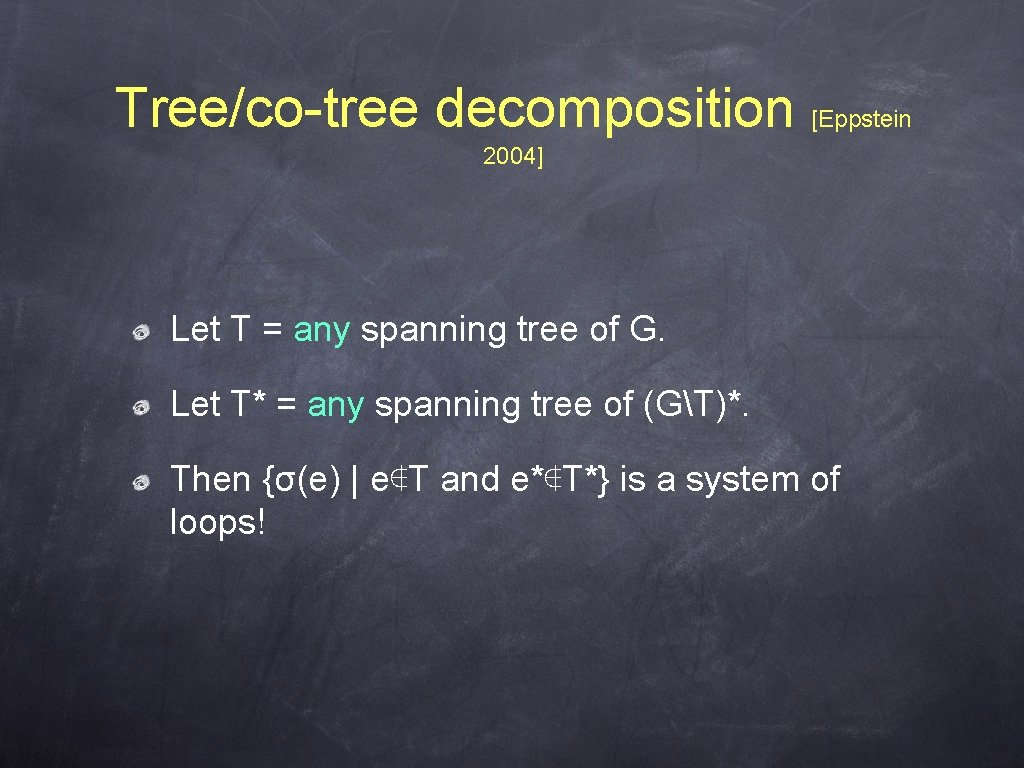Tree/co-tree decomposition [Eppstein 2004] Let T = any spanning tree of G. Let T* = any spanning tree of (GT)*. Then {σ(e) | e∉T and e*∉T*} is a system of loops!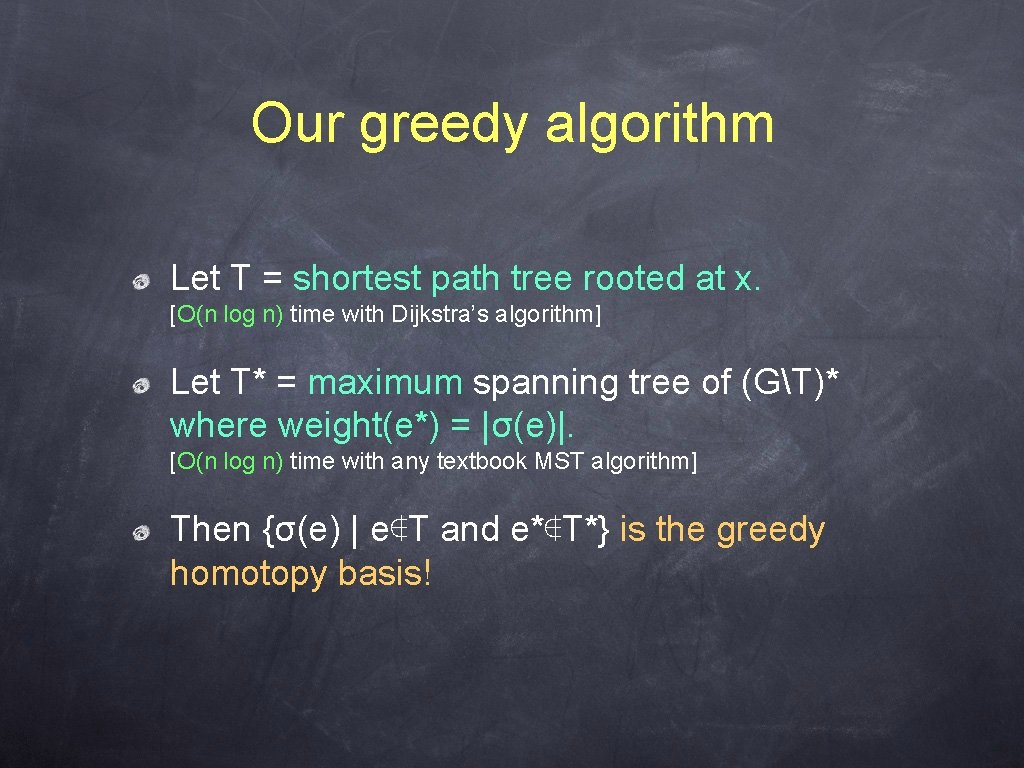Our greedy algorithm Let T = shortest path tree rooted at x. [O(n log n) time with Dijkstra’s algorithm] Let T* = maximum spanning tree of (GT)* where weight(e*) = |σ(e)|. [O(n log n) time with any textbook MST algorithm] Then {σ(e) | e∉T and e*∉T*} is the greedy homotopy basis!Extensions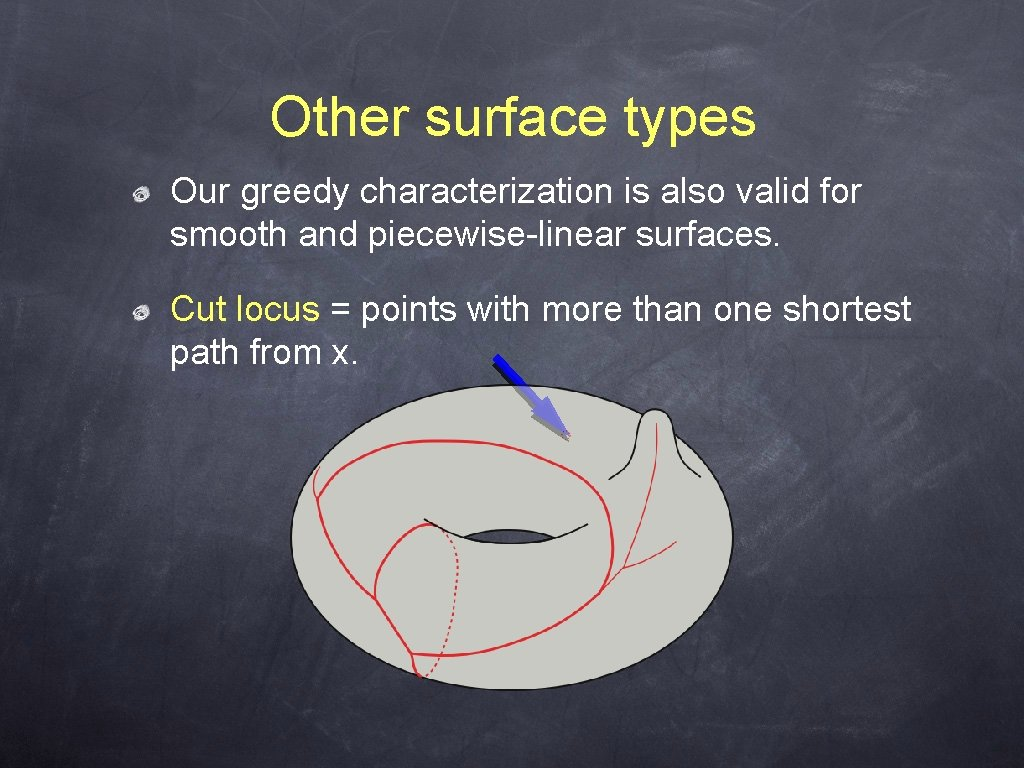Other surface types Our greedy characterization is also valid for smooth and piecewise-linear surfaces. Cut locus = points with more than one shortest path from x.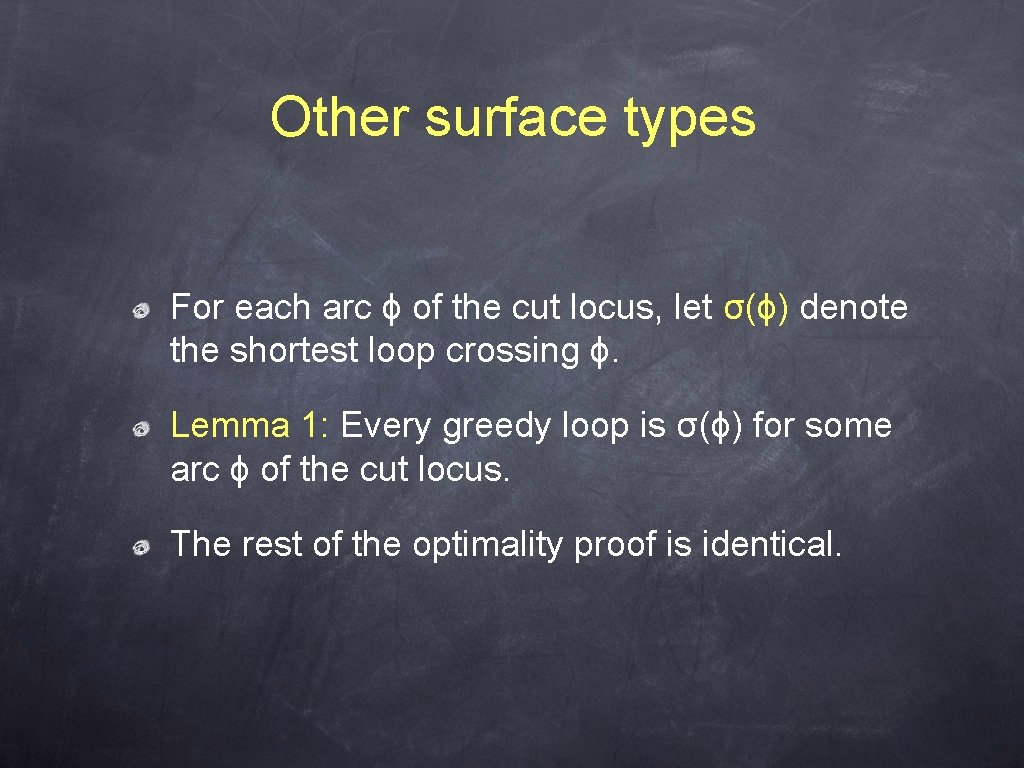Other surface types For each arc ϕ of the cut locus, let σ(ϕ) denote the shortest loop crossing ϕ. Lemma 1: Every greedy loop is σ(ϕ) for some arc ϕ of the cut locus. The rest of the optimality proof is identical.Other surface types Moreover, every greedy loop is σ(ϕ) for some ϕ not in the maximum spanning tree of the cut locus, where weight(ϕ) = |σ(ϕ)|. Once we compute the cut locus, the rest of the algorithm takes O(g log g) time. PL surfaces: O(n 2) time [Chen and Han‘ 96]Thank you!